Dear Family, your Operation Maths guide to Lines and Angles

Dear Family, your Operation Maths guide to Lines and Angles

Category : Uncategorized

Dear Family, given below is a brief guide to understanding the topic of lines and angles as well as some practical suggestions as to how you might support your children’s understanding at home. Also below, are a series of links to digital resources that will help both the children, and you, learn more about lines and angles. The digital resources are organised according to approximate class level:

Understanding Lines and Angles

Line and angles is a strand unit in the Primary Maths Curriculum for 2nd class up. For most people, when they think about angles, they also think about degrees, for example a 90° (ninety degree) angle. Yet in primary school, we don’t introduce degrees, as a way to describe, measure and construct angles, until 5th class. So what are they doing before that?

Initially, children are exploring angles as ‘turns’ i.e. recognising angles in terms of rotation: quarter-turns, half-turns and full turns. The children explore this themselves by turning to show the different turns, in both clockwise (turning right) and anticlockwise (turning left) directions.

In school, the children will also be shown other real-world examples of angles, including angles made by the hands of a clock, by the blades of an open scissors, by a door opening and closing, etc. We also look at angles (or corners/vertices) in 2-D shapes, for example in rectangles and triangles… even the names of these shapes pay homage to the angles that made them what they are today! (triangle = tri (or 3) angle; rectangle = rect (right/proper) angle)

In third class, the children will begin to use the term right angle, as a more mathematically correct way to describe the quarter turn (when movement is involved) or square corner (when there is no movement i.e. the angle is static) that they met in second class. The children will also learn to identify and name a variety of angles: angles less than a right angle (acute angles), angles more than a right angle (obtuse or reflex, more than 2 right angles/a straight angle) and angles equal to 2 right angles (straight angle). Then, in 5th and 6th class, they will begin to use degrees to identify, measure and construct various types of angles. This requires the use of a protractor, from a maths set, and it is not the most obvious or straightforward tool to use, so plenty of practice is required (see video links below in the Digital resources section for 5th and 6th class).

You can’t have an angle without having at least two connecting lines, therefore lines are an integral part of this area of maths. Like angles, not all lines are the same and the children learn to recognise and describe lines as horizontal, vertical, oblique, parallel or perpendicular.

Practical Suggestions for Supporting Children

• Ask your child to teach you about the angles and lines in your home. What different types of lines can be seen? What different types of angles can be seen? Can they name them?
• Line Hunt: ask your child to show you some lines that they can see at home. Ask them to run their finger along the lines so they get a sense of the line’s direction and position.
• Make a right angle finder: From a scrap piece of paper, tear out a large circular shape. Fold the shape in two, and then fold it in two again. The two straight edges/lines meet at a corner to make a right angle. Ask your child:
• ‘Have you heard any other name for this type of angle?’ (square corner, quarter turn)
• ‘Can you find any angles this size in this room? What are they called?’ (right angles)
• ‘Can you find any angles greater than/smaller than right angles in this room?’
• What’s in name? Write out your name in all capital letters. Name the different line types and angle types you can see. Are there any lines of symmetry in the letters? How many lines of symmetry and where? Click here to see some possible answers.
• You gotta hand it to ’em! Look closely at your hand… can you see angles in the lines made by your fingers? Estimate the degrees and then click here to see possible answers.
• Programming If your child does programming, or is interested in trying it out, they could use a free programme such as Scratch to draw various line and angle types.

Digital Resources for Second to Fourth ClassesTurns, Angles and Lines: A series of video lessons from White Rose Maths, including for Year 2,  Describing Turns 1, Describing Turns 2. For Year 3: Right Angles in ShapesCompare Angles, Horizontal & Vertical, Parallel & Perpendicular. For Year 4: Identify Angles and Compare and Order Angles.Lines & Angles: A series of video lessons from Matholia including What are Angles?Right Angles, Identifying Angles, Parallel Lines and Perpendicular LinesKhan Academy – Right Angles: Read through and then answer the practice questions. Afterwards, for something more challenging, look at the Fourth Grade Activities, which explore different types of lines and angles. You can also register for a free Khan Academy account to record your progress and explore other topics/grades.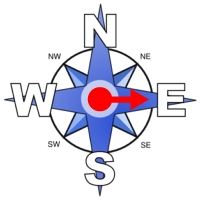Turns on a compass: Compare the start and end positions of the dial and decide how it turned.Turn the man: Explore how many times you need to turn the man to match the images.Turtle Diary: Learn about lines and angles and then take the quizzes! Parallel, perpendicular and intersecting lines; Types of Angles 1; Types of Angles 2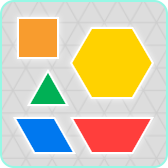Pattern Blocks: Explore the lines and angles that can be made with these interactive pattern blocks. Also includes a protractor feature for measuring the angles.Geoboard: Make lots of different angles and lines using this interactive geoboard, free from the Math Learning Centre.

Acute, Obtuse or Right angle: Answer the quiz questions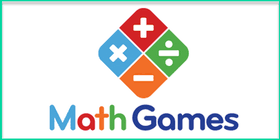Math Games: Identify the parallel, perpendicular and intersecting linesGeometry: a selection of games from ixl.com, including types of angles, obtuse, acute or straight, types of lines. You can do a number of free quizzes each day without having a subscription. (Please note that the class levels given do not always align accurately with the content of the Irish Primary Curriculum.)

Digital Resources for Fifth & Sixth ClassesAngles and Lines: A series of video lessons from White Rose Maths, including Measure with a Protractor, Introduce Angles, Angles in a TriangleKhan Academy – Measuring Angles: A unit of work exploring angles, including how to understand angles, how to measure angles and decomposing angles. Other relevant lessons include this one on the sum of angles in a triangle and this one on the sum of angles in a quadrilateral. You can also register for a free Khan Academy account to record your progress and explore other areas and/or try more difficult material.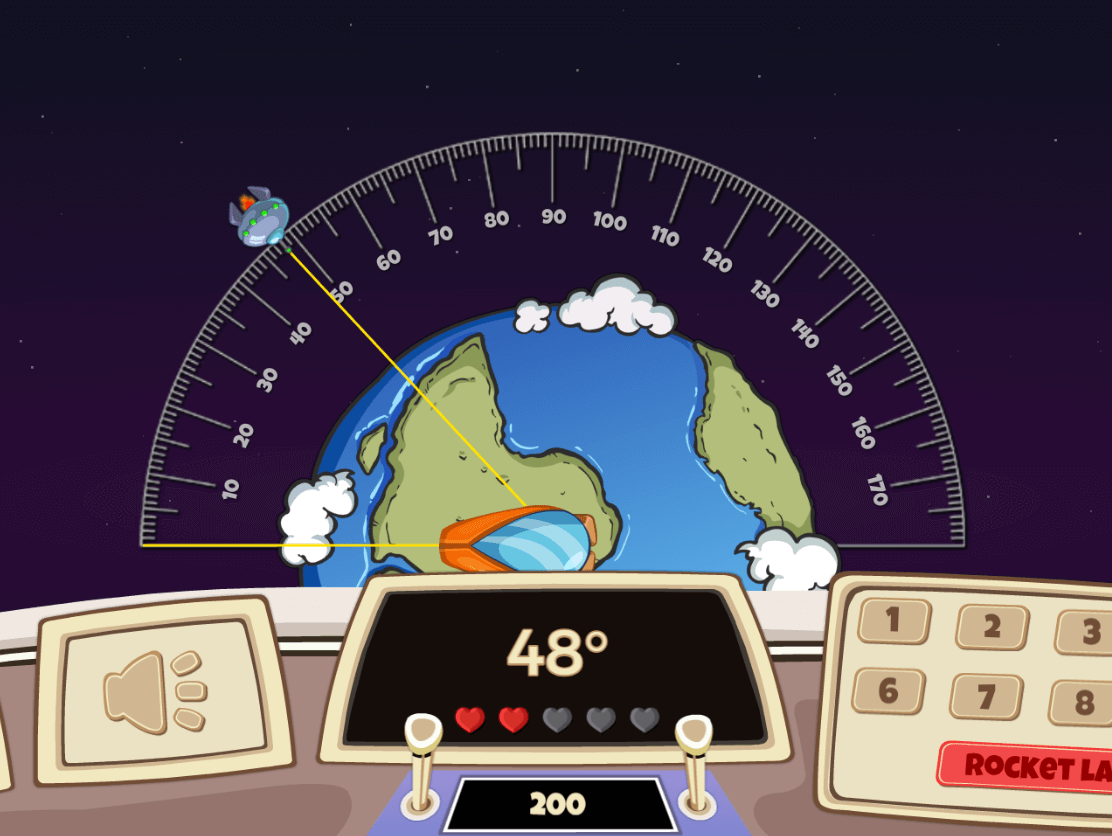Angle Alien Attack: Defend the Earth from an alien invasion using your knowledge of angles. Choose to read the angles from the protractor or estimate them without a protractor.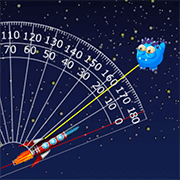Alien Angles: Create a specified angle to destroy the aliens. Challenging, but great for developing the ability to estimate angles. A similar game is Rocket Angles; this time you must estimate and input the measure of the given angle in degrees.Estimating Angles: In this game you must stop the angle size as near as possible to the target measure in degrees.Turtle Diary: Learn about lines and angles and then take the quizzes! Parallel, perpendicular and intersecting lines; Types of Angles 1; Types of Angles 2; Angles in Degrees; Estimating AnglesPattern Blocks: Explore the lines and angles that can be made with these interactive pattern blocks. Also includes a protractor feature for measuring the angles.Geoboard: Make lots of different angles and lines using this interactive geoboard, free from the Math Learning Centre.That Quiz – Angles: This quiz has lots of options, on the left hand side, that can be changed to suit the ability of the child. Ensure that the level is set to 1. Each time do the set 10 questions, if you get 10 or 9 correct go up a level, if not stay at that level. Start with only the “Measure” option on the left-hand side ticked, and when you bring the mouse across the screen, it changes into a transparent protractor. Other options included calculating the value of a missing angle in a triangle, (Triangle), and calculating the value of a missing angle in intersecting lines  (Line) or parallel lines (Parallel).Geometry: a selection of games from ixl.com, including classifying triangles and quadrilaterals. You can do a number of free quizzes each day without having a subscription. (Please note that the class levels given do not always align accurately with the content of the Irish Primary Curriculum.)Math Games: selection of interactive geometry quizzes, that includes lines and angles.

Digging Deeper into … Lines and Angles

Category : Uncategorized

For practical suggestions for families, and links to useful digital resources, to support children learning about the topic of place value, please check out the following post: Dear Family, your Operation Maths Guide to Lines and Angles

Overview:

As can be seen from the overview table below, this topic is initially introduced to the children in 2nd class via “turns” and “square corners” and then develops with increasing complexity in 3rd- 6th classes.

 2nd 3rd 4th 5th 6th Lines vertical horizontal parallel perpendicular oblique Angles Full, half, quarter turns, square corner Right angle Greater/less than a right angle Acute angle Obtuse angle Straight angle Reflex angle Measuring and constructing in degrees Sum of angles in triangle  = 180° Sum of angles in quadrilateral  = 360°

As with every topic in Operation Maths, a CPA approach is also recommended for lines and angles:

• Concrete: allow sufficient time for the children to explore making turns, lines and angles with suitable concrete materials (e.g. the children themselves, lollipop sticks, straws, geo strips, construction materials, real-life examples from the school and home environment)
• Pictorial: activities where the focus is on drawing angles or lines on paper, MWBs etc
• Abstract: the final stage, where the focus is primarily on numbers,  digits  and or letters to represent variables eg given the measure of two angles in a triangle calculate the third angle.

Lines:

Through exploration and activities, it is important that the children realise that:

• A line can be classified and identified according to its position and its relationship to another line.
• A single line can be horizontal, vertical or oblique but a single line cannot on its own be parallel or perpendicular; there must be two or more lines.
• Parallel lines do not all have to be the same length to be parallel.
• Parallel lines do not have to be horizontal or vertical, they can also be oblique.
• Perpendicular lines do not have to have a horizontal and vertical line, (again they can be oblique) but there must be at least one right angle where the two lines meet.

Lines can be drawn on the Operation Maths MWBs and then rotated to reinforce this point.
It is also worth noting that in maths, when we use the word “line”, it should be assumed that this is always straight; only if the word curved is given should it be assumed otherwise.

Angles

In order for the children to recognise angles in terms of rotation, it is preferable initially, for the children to investigate the angles in their environment that are dynamic, (where the angle can be easily made bigger or smaller by increasing or decreasing the distance between the two lines) e.g. a door opening and closing, a scissors cutting paper, the angles made by the hands of a clock. The children can then proceed to examine static angles (where the angle is fixed) e.g. in 2-D shapes or 3D objects.Operation Maths, Pupils’ Book 3: on the digital book, click on the icon on the bottom right to access a “Ready to go” activity.

In second class (and revised at the start of third class), the concept of rotation of an angle is taught through the terms quarter-turn, half-turn and full turn. Ideally, this should be introduced concretely by getting the children themselves to do half-turns and quarter-turns, and to turn in clockwise and anticlockwise directions:

• In the classroom, the children start facing the board/front of room and make half/full/quarter turns to left/right as directed by the teacher.
• Repeat, but this time with different starting points
• Repeat, but this time after the teacher gives the directions the children must say where they will be facing, before they do the actual turn. The children could also record their predictions quickly on their Operation Maths MWBs

The children will be also be asked to identify 90º angles as square corners (2nd class) or right angles (3rd class). This will also be reinforced as part of the 2D shapes chapter. The children can be asked how they might decide if a corner/vertex of a shape is a square corner/right angle. Prior to the introduction of the protractor, something as simple as a corner torn from a piece of paper would suffice as an instrument with which to measure these angles.

The children should be enabled to classify angles according to the criteria appropriate to their class level (see table above). In particular, the ability to identify angles as acute (or less than a right angle), obtuse (or greater than a right angle) or reflex will greatly help the children, to later, estimate the measure of the angle in degrees, and to accurately measure and construct angles when they encounter this in 5th and 6th classes.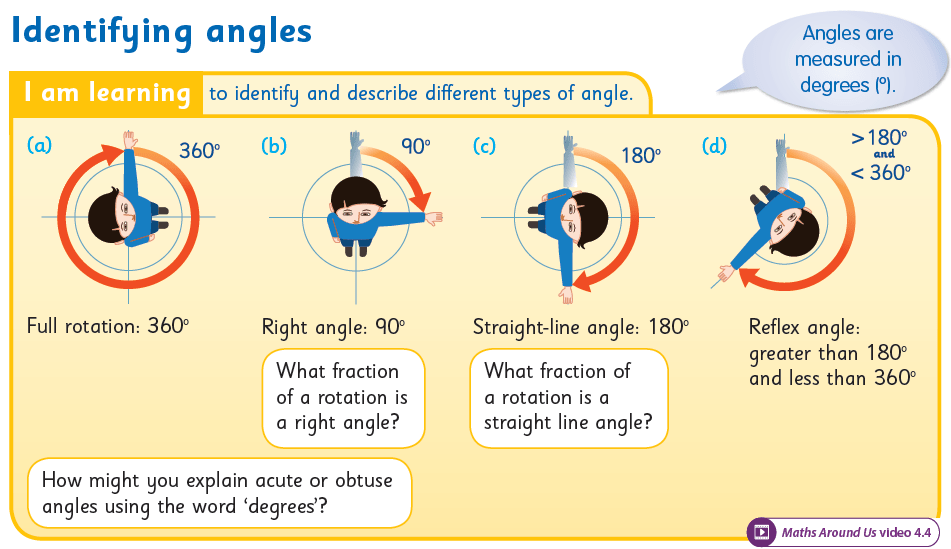Operation Maths, Pupils’ Book 5

It is important to constantly reinforce the children’s understanding of what an angle actually is, i.e. an amount of turn and that this can be represented by two adjoining lines, one showing the starting position, the other showing the point after the turn. Return to concrete examples if necessary; the children stretch out two arms in front and, leaving one arm in original position, they move other arm a certain amount (90 º, 180º etc). This could also be repeated using geostrips, connected at one end using a brass clip, so as to be able to move one of the ‘arms’. Such concrete experiences also link well to measuring using a protractor; the original arm is the ‘base’ line.

Measuring and constructing angles (5th & 6th classes)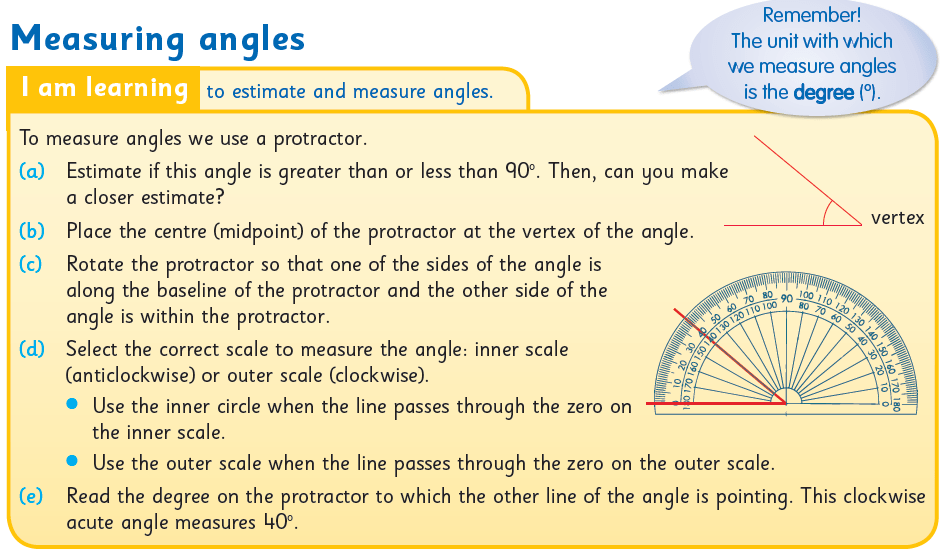Operation Maths, Pupils’ Book 5

Using degrees to describe angles is introduced in 5th class, which develops to include measuring and constructing angles using degrees. This necessitates the use of a protractor for the first time, which in itself can lead to difficulties. The child may be unsure where exactly to place the protractor; this can come from a lack of understanding of what an angle actually is and where the angle actually is. Also, a child can be uncertain of which scale to use to measure the size of the angle eg for an acute angle measuring 45º, the child writes down 135º.

To reduce the likelihood of this arising you can ask some/all of the following questions:

• What important tips would you give to a person about using a protractor?
• How do you know which scale to use on the protractor?
• What type of angle is this? How do you know? (To save time, they can write A, O or Re for Acute, Obtuse or Reflex).
• Estimate the measure of the angle to the nearest 10º. Is your estimate/measurement sensible? Why?
• How can you use what you know about acute and obtuse angles to check your measurement?

You can also watch some of the tutorial videos for using a protractor on the internet, such as the one below, for example. These videos can be a very visual way of demonstrating this skill. See also the list of digital resources for 5th and 6th classes on the following post: Dear Family, your Operation Maths Guide to Lines and Angles.

Angles is another area where it is important for the child to check the reasonableness of the answer. First, the child needs to identify whether the angle is acute or obtuse. Then, if measuring an acute angle, the measurement must be less than 90º. If it isn’t, then the correct scale wasn’t used.

Lines and Angles all around us

It is a given that lines and angles are all around us, although children may often be oblivious to the examples! Again, appropriate to each class level, the children should be encouraged to identify different types of lines and angles in their classroom, school and home. Enrich your own classroom space with lines and angles by labeling the line types in the room and the measure of angles of the open door. Make it personal by relating this topic to the children themselves and to the geometry in their names. Incorporate lines and angles into your visual art lessons (see also image below). Operation Maths 4 and 5 users can show the Maths Around Us video to their class, accessible on www.edcolearning.ie. For more ideas, check out this Lines & Angles board on Pinterest.Operation Maths, Pupils’ Book 4